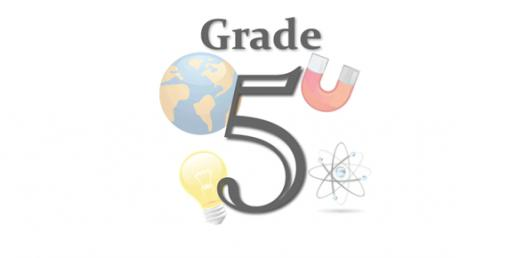5th Grade Science Unit 14 Sound Quiz

8 Questions | Total Attempts: 185SettingsSound is a result of vibrations; these vibrations are what we hear. Unit 14 covers what sound is and how it is produces. The quiz below is designed to help you understand the previous topic by giving you multiple answer questions Give it a try and see what you did not understand

• 1.
What sets sound waves in motion?
• A.

Vibrations

• B.

Amplitude

• C.

Frequency

• D.

Sound

• 2.
What types of wave carry sound energy foward?
• A.

Longitudinal Waves

• B.

Ocean Waves

• C.

Parallel Waves

• D.

Compressed Waves

• 3.
How would you describe the movement of a wave?
• A.

Up and Down

• B.

Side to Side

• C.

Backwards

• D.

Straight

• 4.
How would you describe the movement of a compression wave?
• A.

Straight; Vibrations

• B.

Back and Forth; Vibrations

• C.

Backwards; Vibrations

• D.

Side to Side; Vibrations

• 5.
What does frequency measure?
• A.

How high or low something moves

• B.

How slow something moves

• C.

The number of Vibrations

• D.

How fast something moves

• 6.
Describe Pitch
• A.

A Sound

• B.

A vibration

• C.

How fast something goes

• D.

How High or Low something is

• 7.
What is another word for volume?
• A.

Loudness

• B.

Fastest

• C.

Highest

• D.

Lowest

• 8.
Which one do you like?
• A.

Option 1

• B.

Option 2

• C.

Option 3

• D.

Option 4

Related TopicsBack to top